Language:
Home > News > 4.senior engineer recommend >

## Jumping Off a Building With Bubble Wrap

Jumping Off a Building With Bubble Wrap
Issue Time:2013-05-06
 Editor’s Note: This is a theoretical discussion. We in no way recommend that you try this. In fact, we urge you not to.This was on Reddit:How much bubble wrap would you need to wrap yourself in if you wanted to jump out of a first story window and survive?Why would anyone ask a question like this? Why would I even attempt to answer it? It’s what I do, that’s why. I serve the Interwebs. Perhaps someone in the Reddit comments has already answered this – but I shall proceed anyway.Before I start, I would like to change the question. I am pretty sure you can jump out of a first story window without any bubble wrap. Here I amassuming first story means second story window (or one floor above the ground). Really, this shouldn’t be too difficult to jump from this high. Here is my dangerous jumping calculator. Essentially, the important thing is how far do you travel while stopping. It can be done.The modified question will be: How much bubble wrap do you need to survive jumping out of the 6th floor of a building? Let me randomly say thisis a height of 20 meters.Where would you start with a question like this? Well, first, we need some bubble wrap. What properties can I even measure from bubble wrap?How thick is bubble wrap?Yes, there are many types of bubble wrap, but here is a stack of the stuff I used.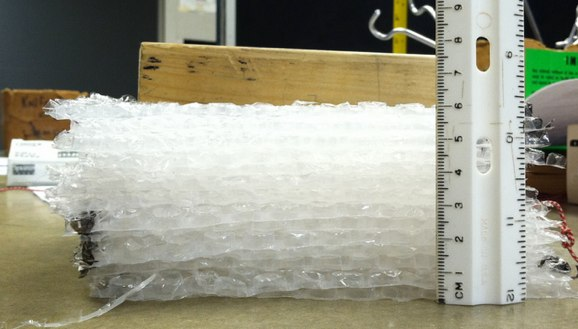To get the thickness, I will make a plot of the height of the stack vs. the number of sheets.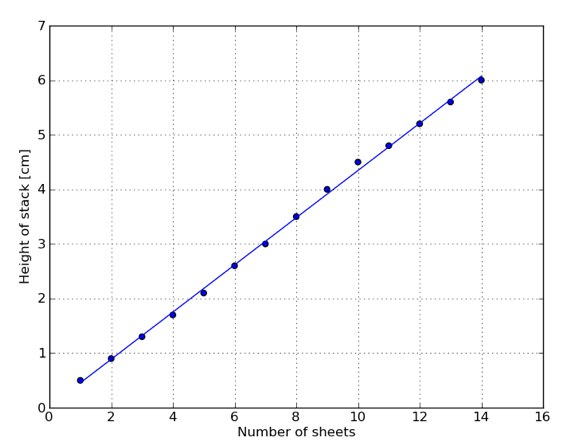The slope of this linear fitting equation is 0.432 cm/sheet. So I will go with this for the thickness of one sheet.What is the density of bubble wrap?Not sure if I will need this, but here it is anyway. I cut the sheets into rectangles (for a reason you will see shortly) that had dimensions of 8.8 cm by 14.3 cm. From above, the height is 0.432 cm. This gives a volume per sheet of 54.3 cm3. To find the mass, I added the stack (one sheet at a time) on a balance. Here is the mass per number of sheets with a linear fit.This line has a slope of 0.922 grams/sheet. So the mass of 1 sheet is about 0.922 grams. From this, I get a bubble wrap density of 0.017 g/cm3.Note that this includes the buoyancy of the bubble wrap, so it isn’t the real density. This ok, since I will be looking at these in air anyway.How springy is bubble wrap?As you push on bubble wrap, it compresses. Does it act like a spring? I don’t know. Here is what I am going to do. I will take my stack of 14 sheets of bubble wrap and measure the height of the stack as I add more mass on top. Here is a picture.If I think about the forces on the mass on top of the stack, I could draw the following force diagram: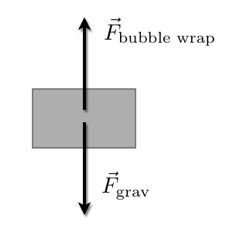Since the masses are in equilibrium, the magnitude of the force from the bubble wrap must be equal to the magnitude of the gravitational force. This gives me a way to easily determine the “spring” force from the bubble wrap. If the bubble wrap acts like a spring, then the force it exerts on the masses should be proportional to the amount the wrap is compressed. If I call the compression amount s, then this would be: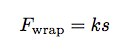Where k is the spring constant. So, here is a plot of force vs. compression.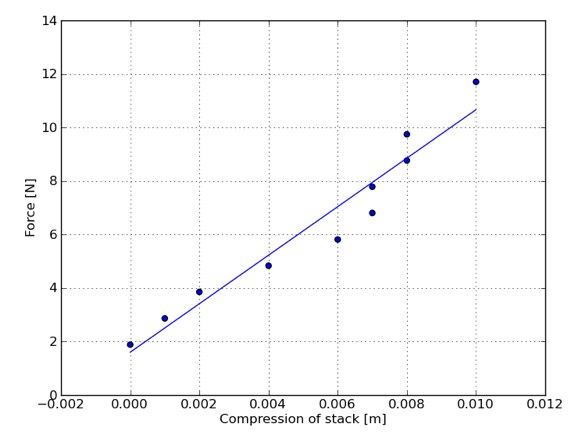The slope of this line is 906 N/m, so that is the effective spring constant for this particular stack. Oh, notice that it looks fairly linear too (that is nice). So, you might think I could just use this to model a collision with a body wrapped in bubbles now, right? Not so fast. What if I made the stack twice as high? Would it have the same spring constant? Not likely. Why? Think of each sheet as a separate spring. All of these sheets have the same force pushing down on them (if I assume the weight of the sheets is small compared to the force) and so they will compress the same amount. If I have 10 sheets that all compress 0.1 cm, the total compression for the stack would be 1 cm (10*0.1 cm).The result is that the bigger the stack, the lower the effective spring constant Also, if I have a larger bubble wrap sheet, there will be more “springs” next to each other to push up on the weights. If I doubled the area of the sheet, the stack would only compress by half as much. So, a larger sheet makes a larger effective spring constant. Maybe you can see that what I really need is Young’s modulus for bubble wrap and not thespring constant of an individual sheet.Young’s modulus is a way to characterize a material that is independent of the dimensions of that material. It is defined as: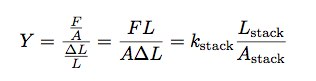Using the data from above, I get a Young’s modulus for bubble wrap with a value of 4319 N/m2.With this, I can find the effective spring constant of any amount of bubble wrap.JumpingIt isn’t the jumping that is dangerous, its the landing. The best way to estimate the safety of a landing is to look at the acceleration. Fortunately, Idon’t need to collect experimental data on the maximum acceleration a body can take, NASA already did this. Here is essentially what they came up with (from the wikipedia page on g-tolerance):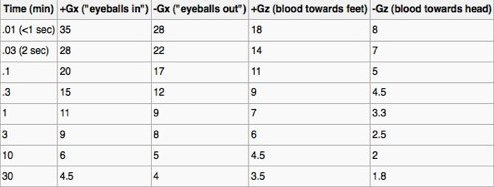From this, you can see a normal body can withstand the greatest accelerations in “eyeballs in” position. This is the orientation such that theacceleration would “push” the eyeballs into the head. In the case of jumping, this means landing on your back.I would normally start with my dangerous jumping calculator. However, there is a problem. The previous calculation determined the acceleration of the lander assuming a constant acceleration. If I am going to model bubble wrap as a spring, then the acceleration would change has the jumper stops. Here is a force diagram of the jumper while stopping:In terms of forces and acceleration, I can write (now just in they-direction):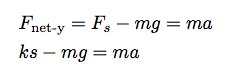So the acceleration depends on the value of the spring constant as well as the distance the spring (bubble wrap) is compressed. I don’t know either of those values. Let me get another expression for the spring compression. Suppose I take the jumper, the Earth and the bubble wrap (spring) as one system. In that case, I can write the work energy principle for the jumper starting at a height h above the ground and ending with the spring compressed.Just to be clear, the jumper velocity (and thus kinetic energy) of the jumper at the top and the bottom are both zero. The gravitational potentialenergy is mgy and the spring potential energy is (1/2)mv2. I now have two expressions with both k and s in them. This will let me solve for k: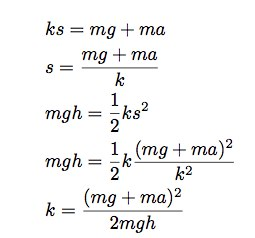Just to be clear, I am putting the maximum acceleration in for a. Also, I have made the assumption that the stopping distance (s) is smallcompared to the jumping height. But the expression looks ok.Let me go head and get an expression for k. Here are my starting values.m = 70 kg. I am assuming the total mass of the bubble wrap is small compared to the mass of the jumper. I can check this  assumption later. a = 300 m/s2 (assuming the collision is   less that 1 second – should be a valid assumption). h = 20 meters (as stated above). This gives a spring constant of 1.7 x 104 N/m.How much bubble wrap?Now that I know the spring constant needed to stop the jumper, I am one step closer to determining how many layers of bubble wrap would be needed. There is one thing I need to estimate first – the area of contact between the ground and the bubble wrap. I know this area should actually change during the collision – so I am just going to estimate it. Suppose the contact makes a square about 0.75 meters on a side. This would give an area of 0.56 m2.I know Young’ modulus for the bubble wrap, so I can find the spring constant as:Here L is the thickness of the bubble wrap. Solving for L: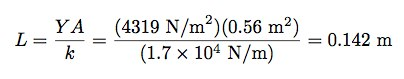With a sheet thickness of 0.432 cm/sheet, you would need (14.2 cm)/(0.432cm/sheet) = 39 sheets. That seems low, but that’s what I get.How much bubble wrap?If I need 39 layers of bubble wrap, how much total would that be? Let me assume that it wraps around the jumper to make a cylindrical shape. Here is sketch.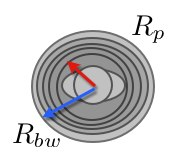Looking down on a person, the person is about a cylinder with a radius of 0.3 meters (just a guess). If the bubble wrap cylinder extends another 0.142 meters, then what is the volume of bubble wrap? Oh, I guess I have to have a person height of about 1.6 meters (another guess). This would give a bubble wrap volume of:Good thing I already calculated the density of bubble wrap. This gives a mass of 9 kg. Not too bad, but this technically would change the amount of bubble wrap needed to land. Just for safety, maybe I would add a couple more layers.What about the size of this bubble wrap falling person? Would this change the air resistance on the person? Certainly. Would it change it enough to matter? I am going to guess: no. When falling from only 20 meters, the falling person probably won’t reach terminal velocity. Oh, don’t believe me? That is ok, I don’t really believe myself either. How about a quick python calculation.Here I will use the following model for the air resistance (as I always do):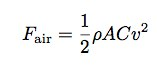Where ρ is the density of air, A is the cross sectional area, and C is the drag coefficient for a cylinder. In this case I will assume the cylinder is falling with the axis of the cylinder parallel to the ground (so the person would land on the back). In this case the cross sectional area would be L*2R. I will use a drag coefficient for the cylinder with a value of 1.05.I will skip the details of the numerical model, but here is a plot of a falling cylinder both with and without air resistance from 20 meters.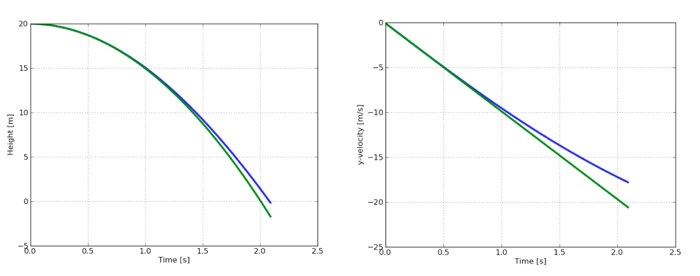Ok, maybe I was wrong. The cylinder with air resistance ends up with a slightly lower speed (17.8 m/s instead of about 20 m/s). Should I redo thecalculations? No, just count it as a safety factor.Final AnswerI am going to go with 39 layers of bubble wrap. Should you actually do this? No. Don’t do this. Well, I guess you could do this with a dummy orsomething.One more quick question. I wonder how much bubble wrap you would need to survive jumping out of a plane. You might not need too much more since all that bubble wrap would also slow your terminal speed down.In the end, maybe you shouldn’t pop that bubble wrap. It might be useful someday. (WARNING: jumping out of a window is not a good idea – just to be clear)Rhett Allain is an Associate Professor of Physics at Southeastern Louisiana University. He enjoys teaching and talking about physics. Sometimes he takes things apart and can’t put them back together.
Contact Person
Will huang